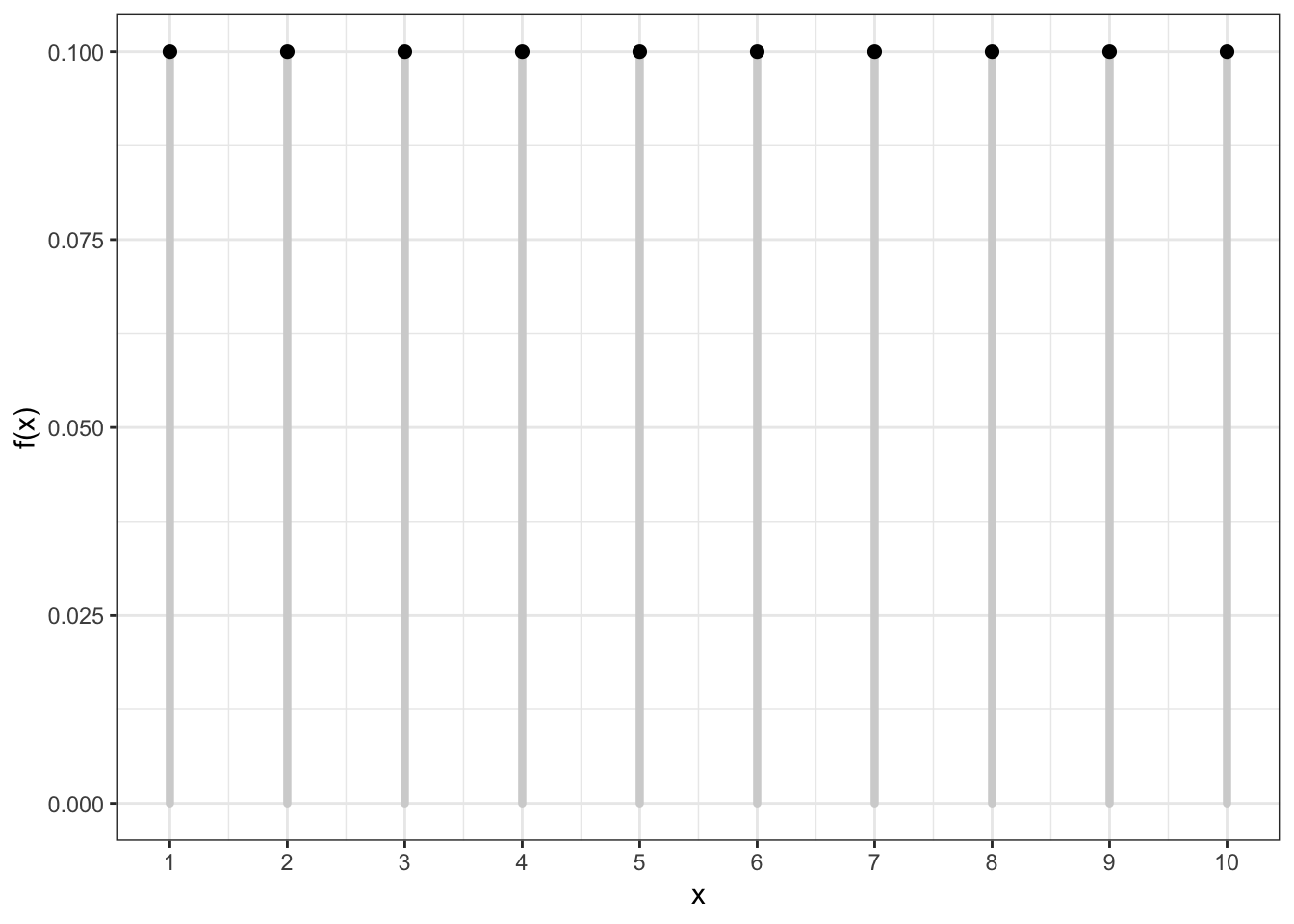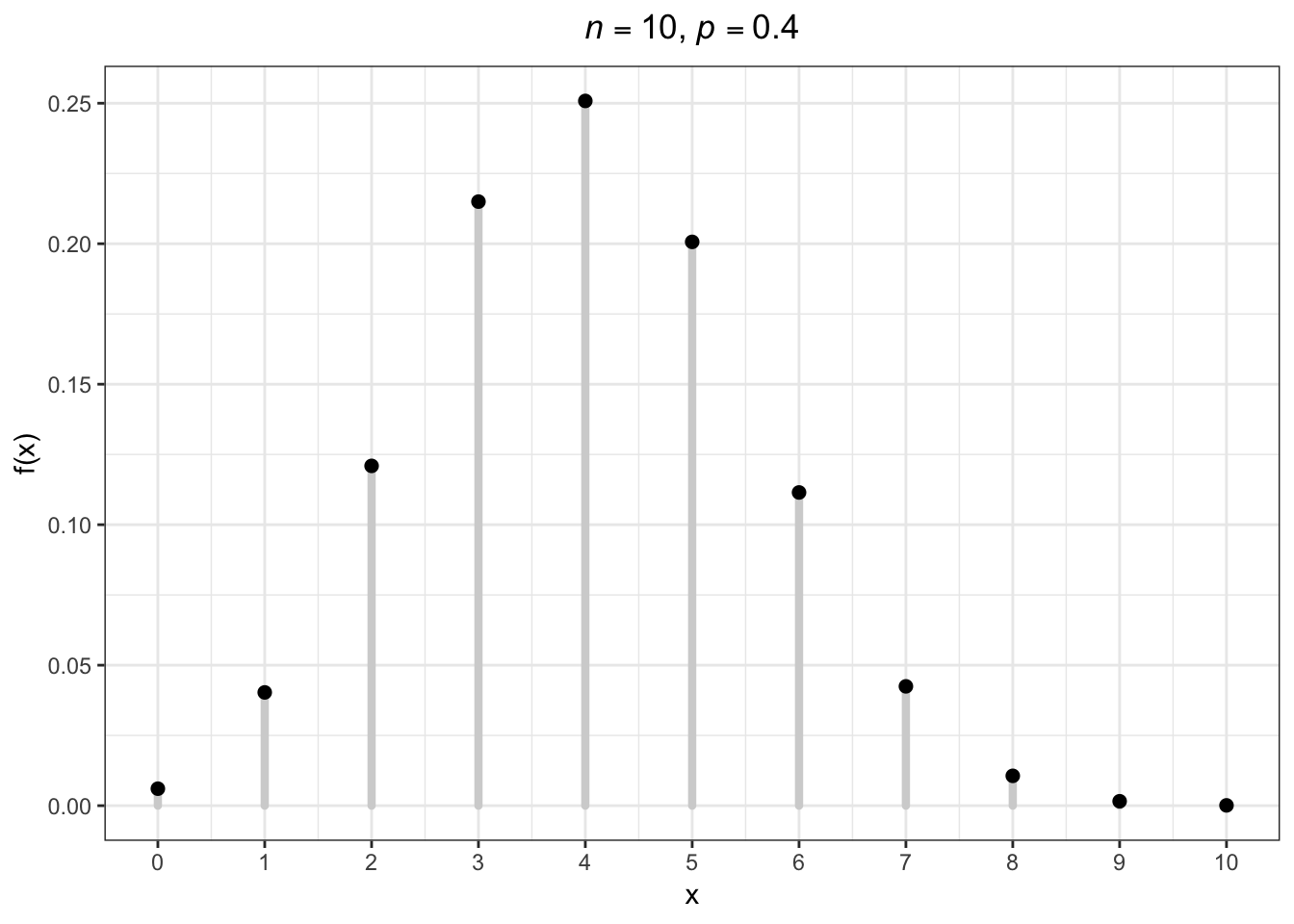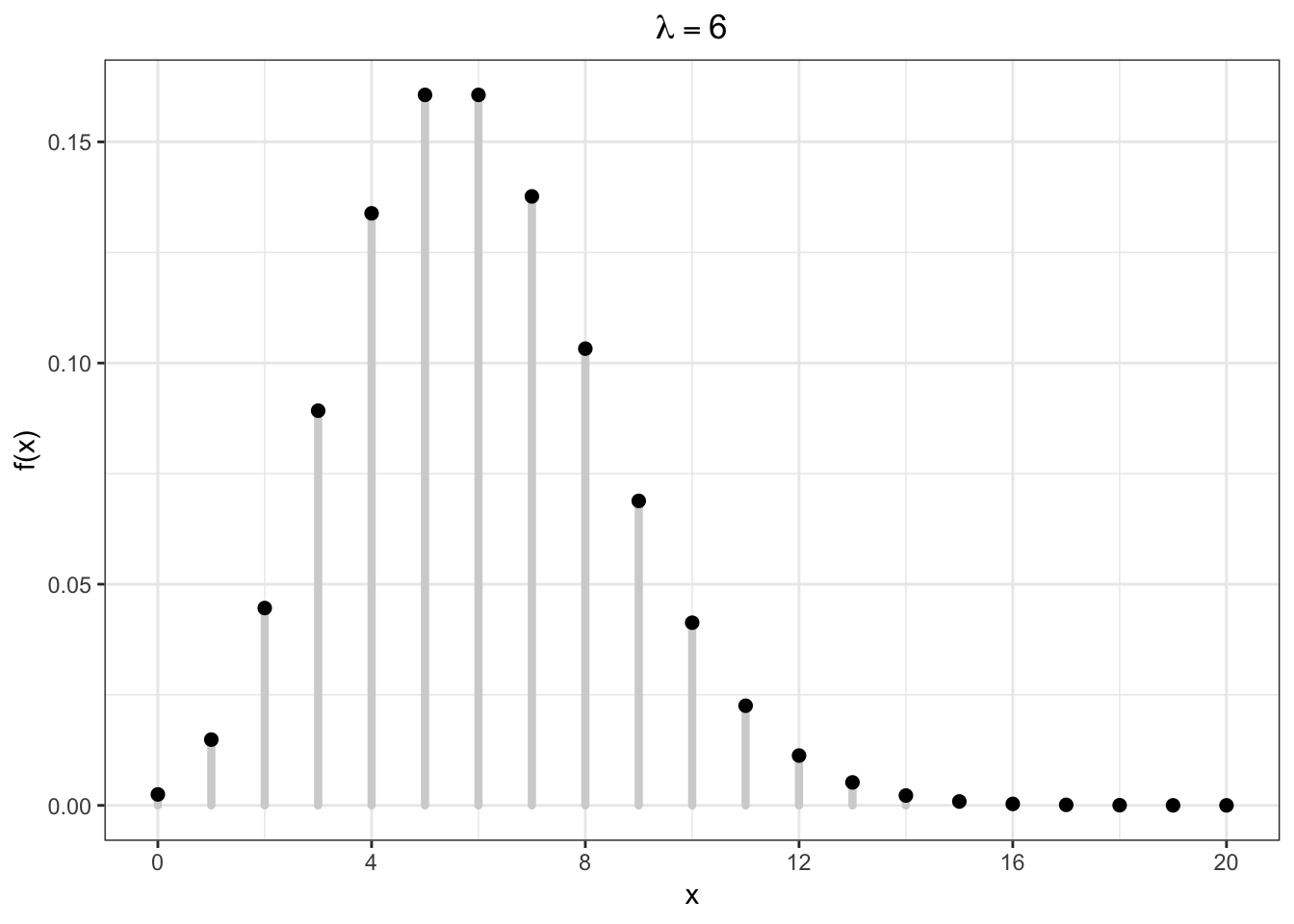13 Discrete RVs

13.1 Uniform (Discrete)

This simple rv distribution assigns equal probabilities to a finite set of values:

$X \sim \mbox{Uniform}\{1, 2, \ldots, n\}$

$\mathcal{R} = \{1, 2, \ldots, n\}$

$f(x; n) = 1/n \mbox{ for } x \in \mathcal{R}$

${\operatorname{E}}[X] = \frac{n+1}{2}, \ {\operatorname{Var}}(X) = \frac{n^2-1}{12}$

13.2 Uniform (Discrete) PMF13.3 Uniform (Discrete) in R

There is no family of functions built into R for this distribution since it is so simple. However, it is possible to generate random values via the sample function:

> n <- 20L
> sample(x=1:n, size=10, replace=TRUE)
  9 15  6  8 13 19 19  1 20 14
>
> x <- sample(x=1:n, size=1e6, replace=TRUE)
> mean(x) - (n+1)/2
 -0.007369
> var(x) - (n^2-1)/12
 0.02429497

13.4 Bernoulli

A single success/failure event, such as heads/tails when flipping a coin or survival/death.

$X \sim \mbox{Bernoulli}(p)$

$\mathcal{R} = \{0, 1\}$

$f(x; p) = p^x (1-p)^{1-x} \mbox{ for } x \in \mathcal{R}$

${\operatorname{E}}[X] = p, \ {\operatorname{Var}}(X) = p(1-p)$

13.5 Binomial

An extension of the Bernoulli distribution to simultaneously considering $$n$$ independent success/failure trials and counting the number of successes.

$X \sim \mbox{Binomial}(n, p)$

$\mathcal{R} = \{0, 1, 2, \ldots, n\}$

$f(x; p) = {n \choose x} p^x (1-p)^{n-x} \mbox{ for } x \in \mathcal{R}$

${\operatorname{E}}[X] = np, \ {\operatorname{Var}}(X) = np(1-p)$

Note that $${n \choose x} = \frac{n!}{x! (n-x)!}$$ is the number of unique ways to choose $$x$$ items from $$n$$ without respect to order.

13.6 Binomial PMF13.7 Binomial in R

> str(dbinom)
function (x, size, prob, log = FALSE)
> str(pbinom)
function (q, size, prob, lower.tail = TRUE, log.p = FALSE)
> str(qbinom)
function (p, size, prob, lower.tail = TRUE, log.p = FALSE)
> str(rbinom)
function (n, size, prob)

13.8 Poisson

Models the number of occurrences of something within a defined time/space period, where the occurrences are independent. Examples: the number of lightning strikes on campus in a given year; the number of emails received on a given day.

$X \sim \mbox{Poisson}(\lambda)$

$\mathcal{R} = \{0, 1, 2, 3, \ldots \}$

$f(x; \lambda) = \frac{\lambda^x e^{-\lambda}}{x!} \mbox{ for } x \in \mathcal{R}$

${\operatorname{E}}[X] = \lambda, \ {\operatorname{Var}}(X) = \lambda$

13.9 Poisson PMF13.10 Poisson in R

> str(dpois)
function (x, lambda, log = FALSE)
> str(ppois)
function (q, lambda, lower.tail = TRUE, log.p = FALSE)
> str(qpois)
function (p, lambda, lower.tail = TRUE, log.p = FALSE)
> str(rpois)
function (n, lambda)